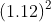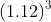Scan QR code or get instant email to install app

Question:

# ABC Company’s dividend growth rate is expected to be 20% for the next three years. After three years, the company’s dividend growth rate will stabilize at 7%. ABC’s last dividend payment was $4 per share. Given that its cost of equity is 12%, the current value of ABC’s stock is closest to: A$119.08.
explanation

1212D1 = 4 x (1.2) = 4.8
D2 = 4.8 x (1.2) = 5.76
D3 = 5.76 x (1.2) = 6.91
D4 = 6.91 x (1.07) = 7.4
Terminal stock price at the end of Year 3 = 7.4 / (0.12 − 0.07) = 147.92
Current stock price = 4.8 / 1.12 + 5.76 /+ 6.91 / (1.12) 3 + 147.92 /= 119.08